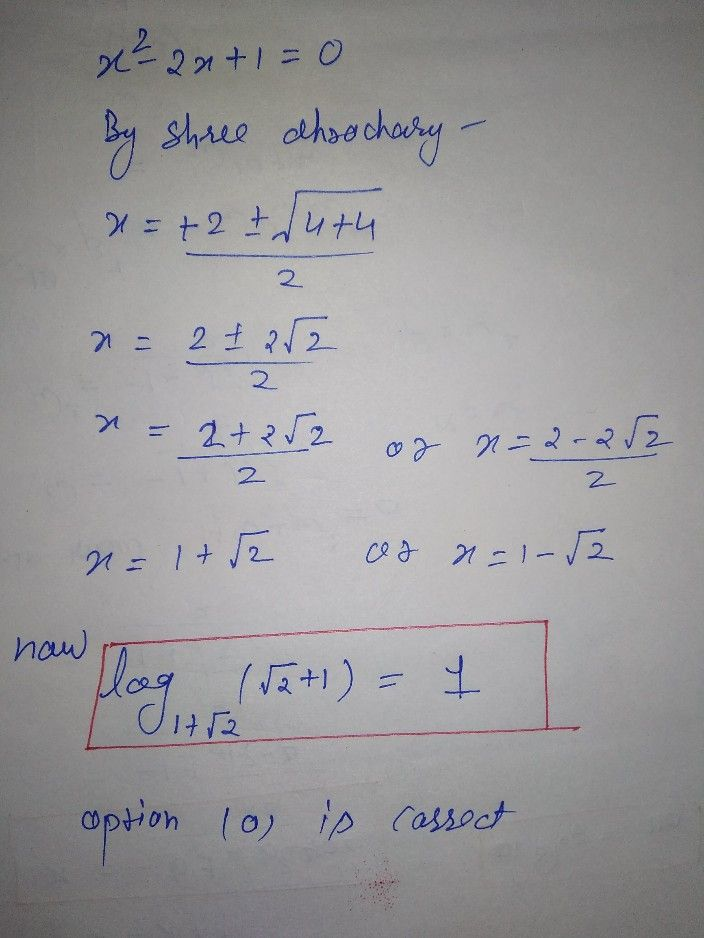Symbol
Problem$2\right)$ $\bar{log\left(x+y+2\right)}$ $x^{2}-2x-1=0$ then $log _{x}\left(\sqrt{2} +1\right)=2$ $\left(a\right)$ $1$ $\left($ (b) $0\right)$ $\sqrt{2} -1$ $\left($ (c) $0$ (d) $-1$ $°°2$
10th-13th grade
Other
Question content
This is the question of logarithm and the answer is 1 but I don't know how?
SolutionQanda teacher - Tarundear Dhruvi shah your question is really good I hope you get your answer
like my profile dear so you can ask directly to me
thanks and have a good eveningStudent
thank u so much ?